## Use arrow notation

We have seen the graphs of the basic reciprocal function and the squared reciprocal function from our study of toolkit functions. Examine these graphs and notice some of their features.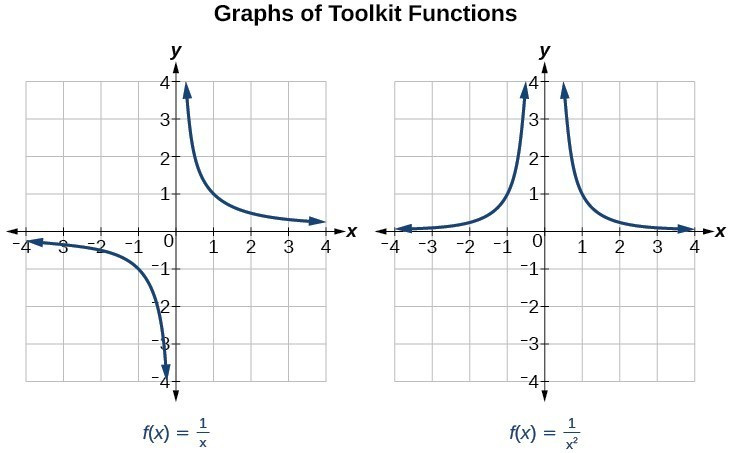Figure 1

Several things are apparent if we examine the graph of $f\left(x\right)=\frac{1}{x}$.

1. On the left branch of the graph, the curve approaches the x-axis $\left(y=0\right) \text{ as } x\to -\infty$.
2. As the graph approaches $x=0$ from the left, the curve drops, but as we approach zero from the right, the curve rises.
3. Finally, on the right branch of the graph, the curves approaches the x-axis $\left(y=0\right) \text{ as } x\to \infty$.

To summarize, we use arrow notation to show that x or $f\left(x\right)$ is approaching a particular value.

Arrow Notation
Symbol Meaning
$x\to {a}^{-}$ x approaches a from the left (< a but close to a)
$x\to {a}^{+}$ x approaches a from the right (> a but close to a)
$x\to \infty\\$ x approaches infinity (x increases without bound)
$x\to -\infty$ x approaches negative infinity (x decreases without bound)
$f\left(x\right)\to \infty$ the output approaches infinity (the output increases without bound)
$f\left(x\right)\to -\infty$ the output approaches negative infinity (the output decreases without bound)
$f\left(x\right)\to a$ the output approaches a

## Local Behavior of $f\left(x\right)=\frac{1}{x}$

Let’s begin by looking at the reciprocal function, $f\left(x\right)=\frac{1}{x}$. We cannot divide by zero, which means the function is undefined at $x=0$; so zero is not in the domain. As the input values approach zero from the left side (becoming very small, negative values), the function values decrease without bound (in other words, they approach negative infinity). We can see this behavior in the table below.

 x –0.1 –0.01 –0.001 –0.0001 $f\left(x\right)=\frac{1}{x}$ –10 –100 –1000 –10,000

We write in arrow notation

$\text{as }x\to {0}^{-},f\left(x\right)\to -\infty$

As the input values approach zero from the right side (becoming very small, positive values), the function values increase without bound (approaching infinity). We can see this behavior in the table below.

 x 0.1 0.01 0.001 0.0001 $f\left(x\right)=\frac{1}{x}$ 10 100 1000 10,000

We write in arrow notation

$\text{As }x\to {0}^{+}, f\left(x\right)\to \infty$.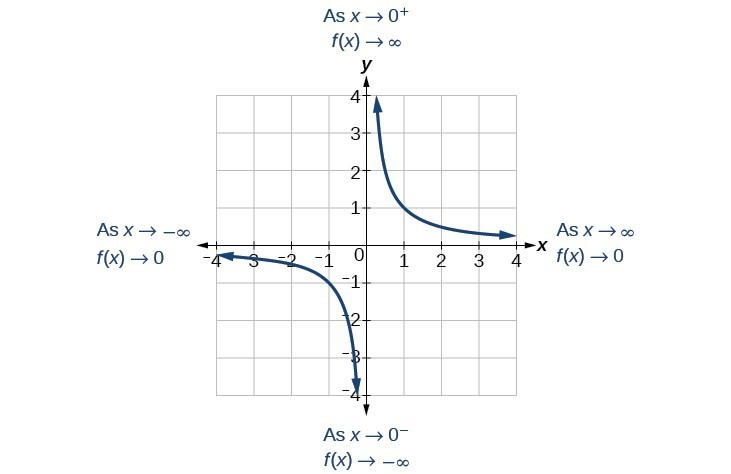Figure 2

This behavior creates a vertical asymptote, which is a vertical line that the graph approaches but never crosses. In this case, the graph is approaching the vertical line = 0 as the input becomes close to zero.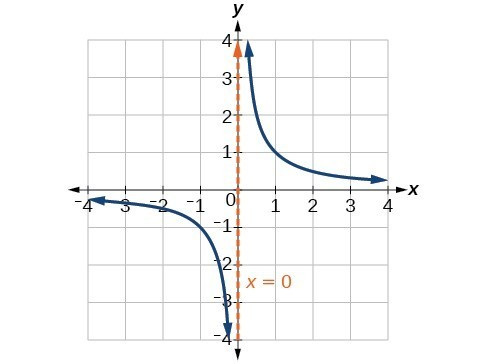Figure 3

### A General Note: Vertical Asymptote

A vertical asymptote of a graph is a vertical line $x=a$ where the graph tends toward positive or negative infinity as the inputs approach a. We write

$\text{As }x\to a,f\left(x\right)\to \infty , \text{or as }x\to a,f\left(x\right)\to -\infty$.

## End Behavior of $f\left(x\right)=\frac{1}{x}$

As the values of x approach infinity, the function values approach 0. As the values of x approach negative infinity, the function values approach 0. Symbolically, using arrow notation

$\text{As }x\to \infty ,f\left(x\right)\to 0,\text{and as }x\to -\infty ,f\left(x\right)\to 0$.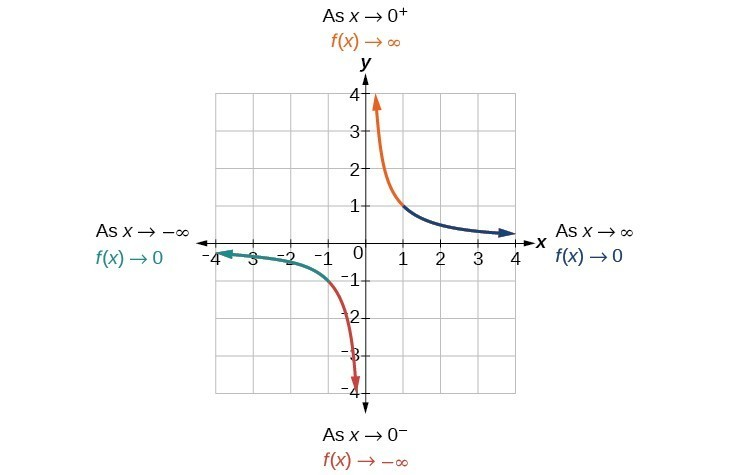Figure 4

Based on this overall behavior and the graph, we can see that the function approaches 0 but never actually reaches 0; it seems to level off as the inputs become large. This behavior creates a horizontal asymptote, a horizontal line that the graph approaches as the input increases or decreases without bound. In this case, the graph is approaching the horizontal line $y=0$.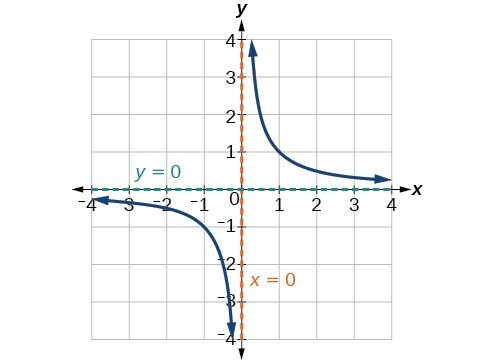Figure 5

### A General Note: Horizontal Asymptote

A horizontal asymptote of a graph is a horizontal line $y=b$ where the graph approaches the line as the inputs increase or decrease without bound. We write

$\text{As }x\to \infty \text{ or }x\to -\infty ,\text{ }f\left(x\right)\to b$.

### Example 1: Using Arrow Notation

Use arrow notation to describe the end behavior and local behavior of the function graphed in Figure 6.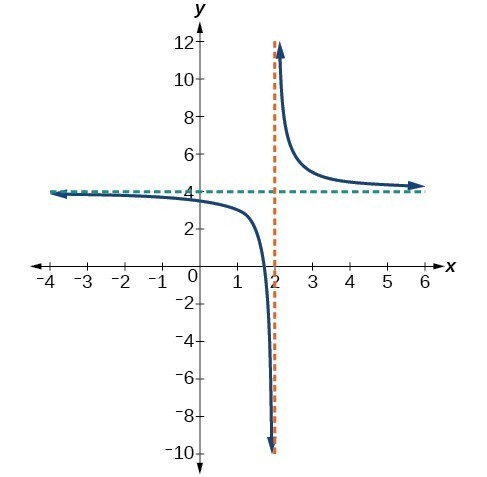Figure 6

### Solution

Notice that the graph is showing a vertical asymptote at $x=2$, which tells us that the function is undefined at $x=2$.

$\text{As }x\to {2}^{-},f\left(x\right)\to -\infty ,\text{ and as }x\to {2}^{+},\text{ }f\left(x\right)\to \infty$.

And as the inputs decrease without bound, the graph appears to be leveling off at output values of 4, indicating a horizontal asymptote at $y=4$. As the inputs increase without bound, the graph levels off at 4.

$\text{As }x\to \infty ,\text{ }f\left(x\right)\to 4\text{ and as }x\to -\infty ,\text{ }f\left(x\right)\to 4$.

### Try It 1

Use arrow notation to describe the end behavior and local behavior for the reciprocal squared function.

Solution

### Example 2: Using Transformations to Graph a Rational Function

Sketch a graph of the reciprocal function shifted two units to the left and up three units. Identify the horizontal and vertical asymptotes of the graph, if any.

### Solution

Shifting the graph left 2 and up 3 would result in the function

$f\left(x\right)=\frac{1}{x+2}+3$

or equivalently, by giving the terms a common denominator,

$f\left(x\right)=\frac{3x+7}{x+2}$

The graph of the shifted function is displayed in Figure 7.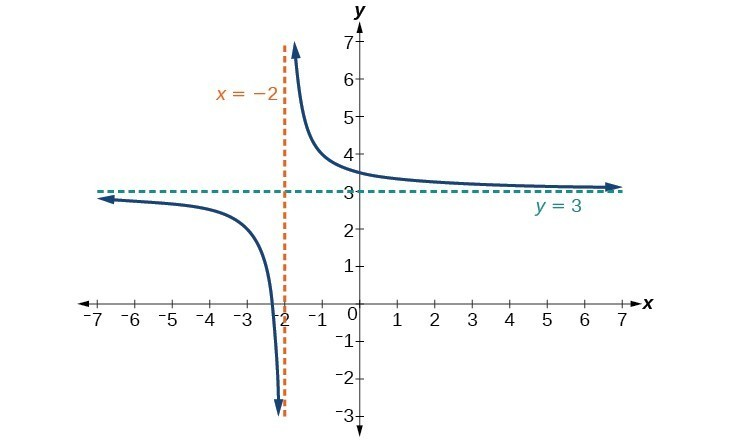Figure 7

Notice that this function is undefined at $x=-2$, and the graph also is showing a vertical asymptote at $x=-2$.

$\text{As }x\to -{2}^{-}, f\left(x\right)\to -\infty ,\text{ and as} x\to -{2}^{+}, f\left(x\right)\to \infty$.

As the inputs increase and decrease without bound, the graph appears to be leveling off at output values of 3, indicating a horizontal asymptote at $y=3$.

$\text{As }x\to \pm \infty , f\left(x\right)\to 3$.

### Analysis of the Solution

Notice that horizontal and vertical asymptotes are shifted left 2 and up 3 along with the function.

### Try It 2

Sketch the graph, and find the horizontal and vertical asymptotes of the reciprocal squared function that has been shifted right 3 units and down 4 units.

Solution